Concurrent Deviation - Correlation & Regression, Business Mathematics & Statistics

# Concurrent Deviation - Correlation & Regression, Business Mathematics & Statistics - Business Mathematics and Statistics - B Com

Coefficient of concurrent deviations :

A very simple and casual method of finding correlation when we are not serious about the magnitude of the two variables is the application of concurrent deviations.

This method involves in attaching a positive sign for a x-value (except the first) if this value is more than the previous value and assigning a negative value if this value is less than the previous value.

This is done for the y-series as well. The deviation in the x-value and the corresponding y-value is known to be concurrent if both the deviations have the same sign.

Denoting the number of concurrent deviation by c and total number of deviations as m (which must be one less than the number of pairs of x and y values), the coefficient of concurrent-deviations is given by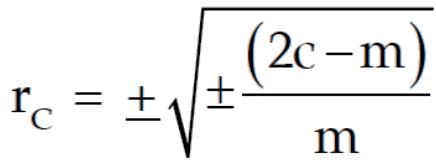If (2c–m) > 0, then we take the positive sign both inside and outside the radical sign and if (2c–m) < 0, we are to consider the negative sign both inside and outside the radical sign.

Like Pearson’s correlation coefficient and Spearman’s rank correlation coefficient, the coefficient of concurrent- deviations also lies between –1 and 1, both inclusive.

That is,

-1 ≤ r ≤ 1

Coefficient of concurrent-deviations - Practice problem

Problem :

Find the coefficient of concurrent deviations from the following data.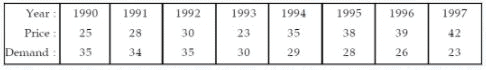Solution :

Computation of Coefficient of Concurrent-Deviations.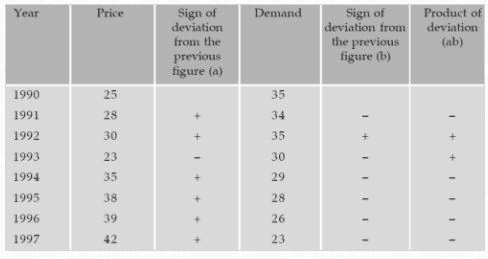In this case,

m  =  number of pairs of deviations

m  =  7

c  =  No. of positive signs in the product of deviation column

c  =  Number of concurrent deviations

c  =  2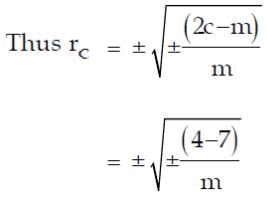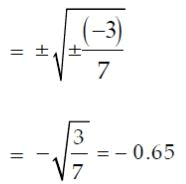Coefficient of concurrent- deviations - Special case

We attach a positive sign for a x-value (except the first) if this value is more than the previous value and assigning a negative value if this value is less than the previous value.

In case, a particular value is equal to the previous value, we have to attach "=" sign to that value.

And we have to do product of deviations as given below.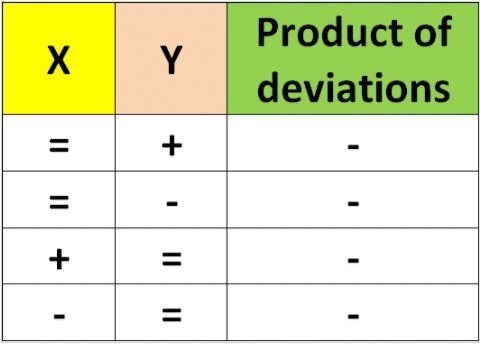Since ( "="  and  "+" ) or ( "="  and  "-" ) are different signs, we have to put negative sign in the column "product of deviations".

Note :

There are some cases when we may find a correlation between two variables although the two variables are not causally related.

This is due to the existence of a third variable which is related to both the variables under consideration.

Such a correlation is known as spurious correlation or non-sense correlation.

As an example, there could be a positive correlation between production of rice and that of iron in India for the last twenty years due to the effect of a third variable time on both these variables.

It is necessary to eliminate the influence of the third variable before computing correlation between the two original variables.

After having gone through the stuff given above, we hope that the students would have understood "Coefficient of concurrent-deviations".

The document Concurrent Deviation - Correlation & Regression, Business Mathematics & Statistics | Business Mathematics and Statistics - B Com is a part of the B Com Course Business Mathematics and Statistics.
All you need of B Com at this link: B Com

115 videos|142 docs

## FAQs on Concurrent Deviation - Correlation & Regression, Business Mathematics & Statistics - Business Mathematics and Statistics - B Com

 1. What is concurrent deviation in correlation and regression?Ans. Concurrent deviation is the difference between the actual value of a dependent variable and the predicted value of that variable using a regression equation. It is also known as the residual or error term. The goal of regression analysis is to minimize the sum of the squared deviations between the predicted and actual values.
 2. How is concurrent deviation calculated?Ans. Concurrent deviation is calculated by subtracting the predicted value of the dependent variable from the actual value of the dependent variable. The resulting difference is the deviation or residual. The sum of the squared deviations is then calculated to determine the total amount of error in the regression equation.
 3. What is the importance of concurrent deviation in regression analysis?Ans. The concurrent deviation is an essential component of regression analysis as it measures the accuracy of the regression equation in predicting the values of the dependent variable. A high level of concurrent deviation indicates that the regression equation is not a good fit for the data, while a low level of concurrent deviation indicates a good fit.
 4. How is concurrent deviation used in business mathematics and statistics?Ans. In business mathematics and statistics, concurrent deviation is used to evaluate the effectiveness of regression models in predicting business outcomes. It is frequently used in financial analysis, marketing research, and other areas of business to identify trends and forecast future performance.
 5. Can concurrent deviation be negative?Ans. Yes, concurrent deviation can be negative. A negative deviation indicates that the predicted value of the dependent variable is greater than the actual value. This can occur when the regression equation overestimates the value of the dependent variable. The sum of the squared deviations is calculated using absolute values, so negative deviations are treated the same as positive deviations in regression analysis.

115 videos|142 docsExplore Courses for B Com examSignup to see your scores go up within 7 days! Learn & Practice with 1000+ FREE Notes, Videos & Tests.
10M+ students study on EduRev
Track your progress, build streaks, highlight & save important lessons and more!
Related Searches

,

,

,

,

,

,

,

,

,

,

,

,

,

,

,

,

,

,

,

,

,

,

,

,

;